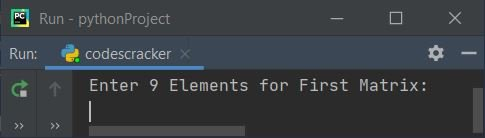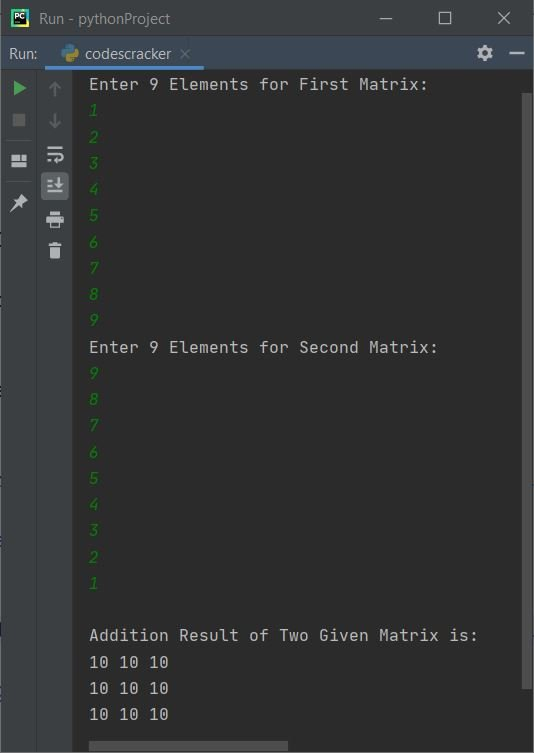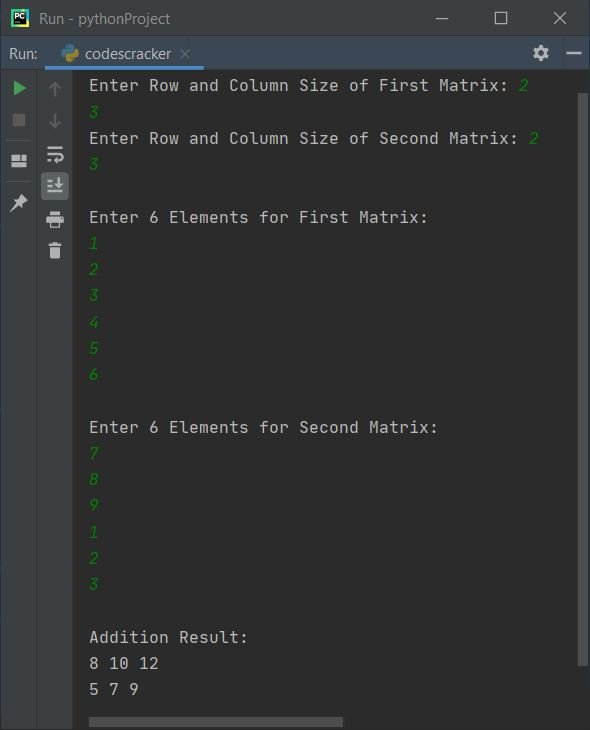# Python Program to Add Two Matrices

In this article, you will learn and get code in Python to add two matrices entered by user at run-time. Here are the list of programs on matrix addition in Python:

• Add Two Matrices of Given Size

Note - Before starting the program, if you're not aware about, how the addition of any two matrices gets defined, refer to Matrix Addition to get every required thing. Now let's start the program.

## Add Two Matrices in Python

This program find and prints the addition result of two given 3*3 matrices. Here 3*3 matrix means, matrix of 3 rows and 3 columns.

```matOne = []
print("Enter 9 Elements for First Matrix: ")
for i in range(3):
matOne.append([])
for j in range(3):
num = int(input())
matOne[i].append(num)

matTwo = []
print("Enter 9 Elements for Second Matrix: ")
for i in range(3):
matTwo.append([])
for j in range(3):
num = int(input())
matTwo[i].append(num)

matThree = []
for i in range(3):
matThree.append([])
for j in range(3):
matThree[i].append(matOne[i][j]+matTwo[i][j])

print("\nAddition Result of Two Given Matrix is:")
for i in range(3):
for j in range(3):
print(matThree[i][j], end=" ")
print()```

Here is the initial output produced by this Python program:Now supply inputs say 1, 2, 3, 4, 5, 6, 7, 8, 9 as nine elements for first and 9, 8, 7, 6, 5, 4, 3, 2, 1 as nine elements for second matrix, to add these two given matrices as shown in the following snapshot:Note - In above program, we've used nested list to apply the thing to perform matrix addition. That is, with following statement:

`matOne = []`

a list named matOne gets defined. And using the following statement:

`matOne.append([])`

Another empty list gets appended to matOne. Now matOne becomes nested list. For example, if user enters the same input as provided in above sample run. then matOne holds those numbers in this way:

`matOne = [[1, 2, 3], [4, 5, 6], [7, 8, 9]]`

## Add Two Matrices of Given Size by User

This program does the same job as of previous program. The only difference is, this program allows user to define the size of matrix along with its elements.

```print("Enter Row and Column Size of First Matrix: ", end="")
rowOne = int(input())
colOne = int(input())
print("Enter Row and Column Size of Second Matrix: ", end="")
rowTwo = int(input())
colTwo = int(input())

if rowOne==rowTwo and colOne==colTwo:
matOne = []
print("\nEnter " +str(rowOne*colOne)+ " Elements for First Matrix: ")
for i in range(rowOne):
matOne.append([])
for j in range(colOne):
num = int(input())
matOne[i].append(num)

matTwo = []
print("\nEnter " +str(rowTwo*colTwo)+ " Elements for Second Matrix: ")
for i in range(rowTwo):
matTwo.append([])
for j in range(colTwo):
num = int(input())
matTwo[i].append(num)

matThree = []
for i in range(rowOne):
matThree.append([])
for j in range(colTwo):
matThree[i].append(matOne[i][j]+matTwo[i][j])

for i in range(rowOne):
for j in range(colOne):
print(matThree[i][j], end=" ")
print()
else:
print("\nDimension Mismatched!")```

Here is its sample run with user input, 2 as row size and 3 as column size of both matrices. Then 1, 2, 3, 4, 5, 6 as six elements for first and 7, 8, 9, 1, 2, 3 as six elements for second matrix:#### Same Program in Other Languages

Python Online Test

« Previous Program Next Program »